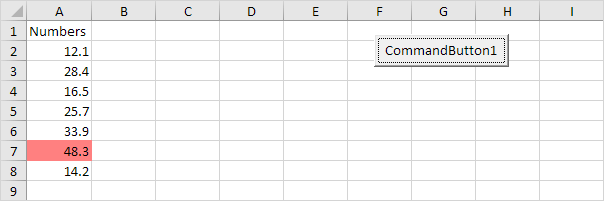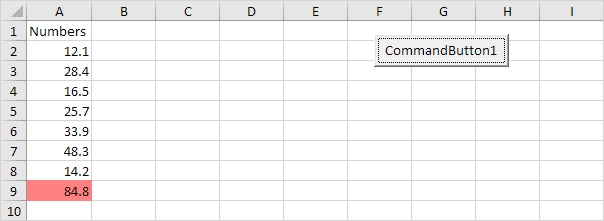# Dynamic Range

Below we will look at a program in Excel VBA that colors the maximum value of a dynamic range.

Situation:

Each time we add a number and we click the command button, we want Excel VBA to color the maximum value of these numbers.Place a command button on your worksheet and add the following code lines:

1. First, we declare one variable and two Range objects. One variable of type Double we call maximum. We call the Range objects rng and cell.

Dim maximum As Double, rng As Range, cell As Range

2. We add the line which changes the background color of all cells to 'No Fill'.

Cells.Interior.ColorIndex = 0

3. We initialize rng with the numbers. We use the CurrentRegion property for this. CurrentRegion is useful when we don't know the exact boundaries of a range in advance.

Set rng = Range("A1").CurrentRegion

4. We initialize maximum with the maximum value of the numbers. We use the worksheet function Max to find the maximum value.

maximum = WorksheetFunction.Max(rng)

5. Finally, we color the maximum value. We use a For Each Next Loop.

For Each cell In rng
If cell.Value = maximum Then cell.Interior.ColorIndex = 22
Next cell

Note: instead of ColorIndex number 22 (red), you can use any ColorIndex number.